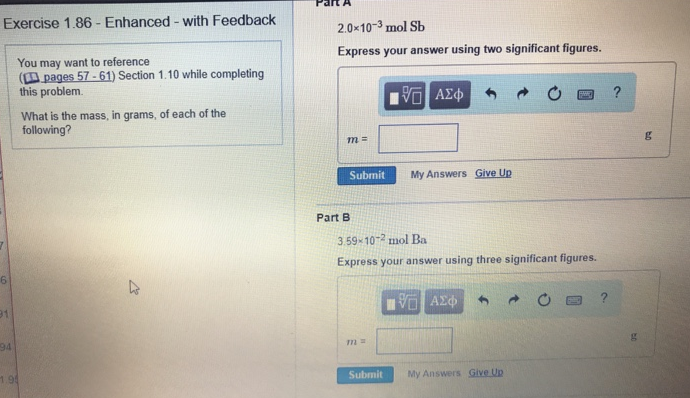# Problem: What is the mass, in grams, of each of the following?Part A2.0 x 10-3 mol Sb Express your answer using two significant figures.  Part B 3.59 x 10-2 mol Ba Express your answer using three significant figures.

###### FREE Expert Solution
83% (180 ratings)###### Problem Details

What is the mass, in grams, of each of the following?

Part A

2.0 x 10-3 mol Sb

Part B

3.59 x 10-2 mol Ba